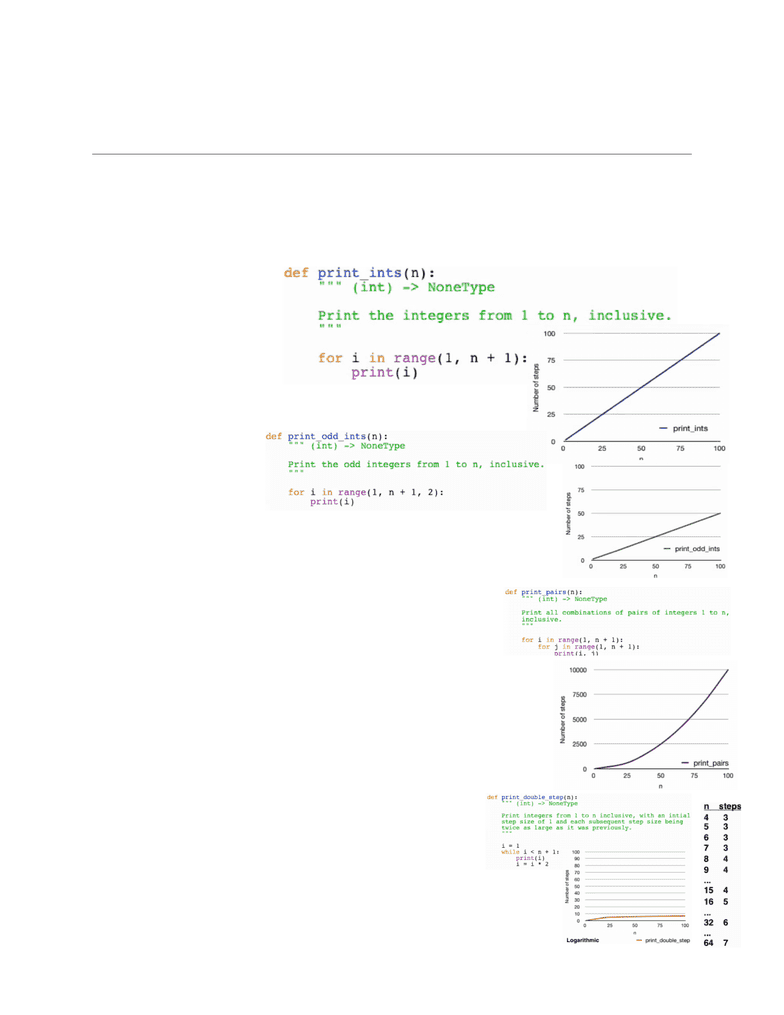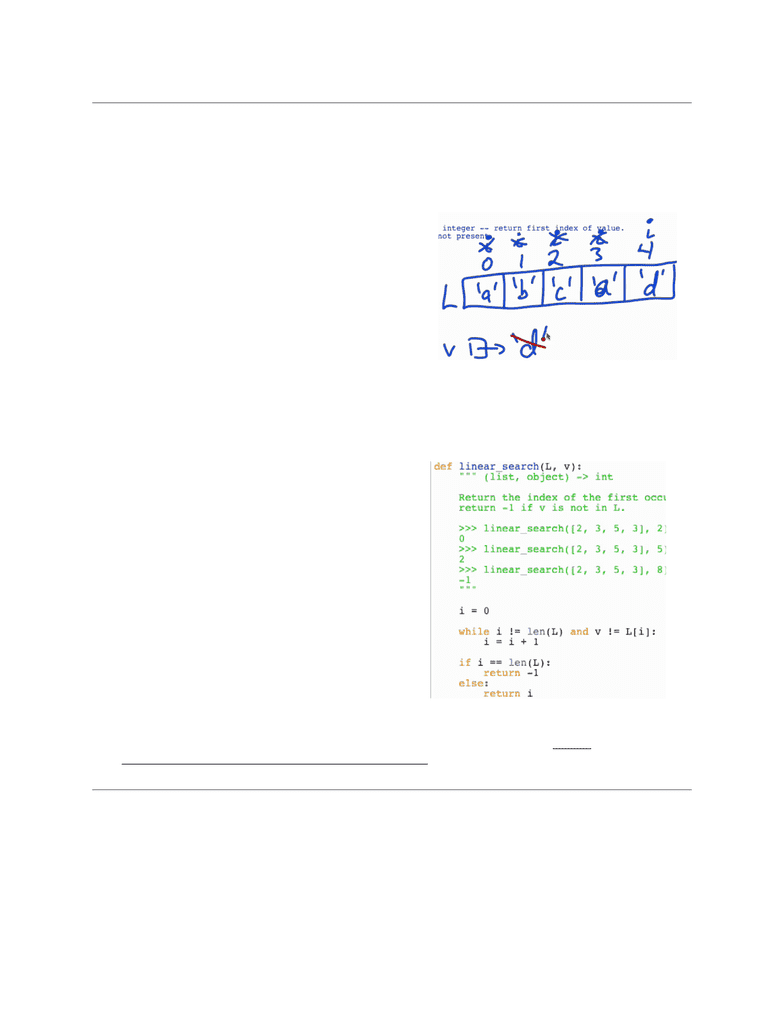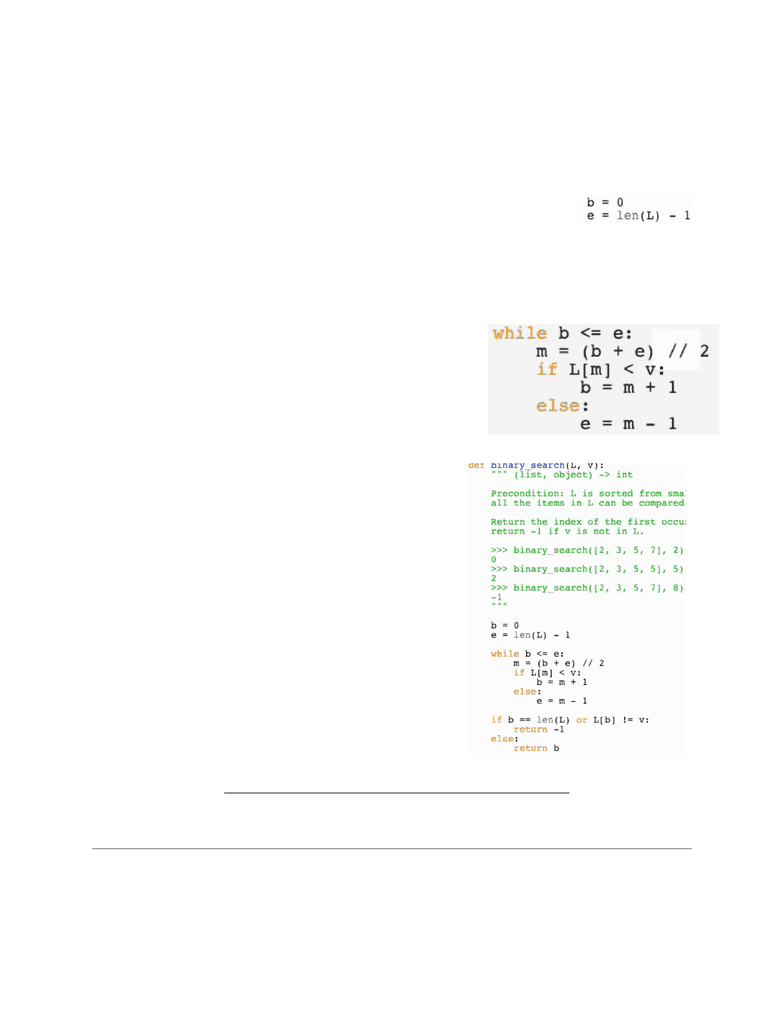Class Notes (1,100,000)
CA (620,000)
UTSC (30,000)
All (10)
Lecture 10

# CSCA08 Lecture 10: Week 10 Notes

Department
Computer Science
Course Code
CSCA08H3
Professor
All
Lecture
10

This preview shows pages 1-2. to view the full 8 pages of the document.Alexander Magony
CSC108H1F 2014
Week 10
Analyzing Algorithms
-Up until now we’ve been concentrating on creating correct functions. Now we’re going to take a look at
the time it takes the the function to execute relative to the size of the input.
Instead of timing it, we will look at the amount of steps it takes.
Consider the following functions:
-print_ints executes the print
function n times, printing
every integer from 1 to n.
As n increases, the
number of steps (in this
case, prints) increases
linearly (eg. 20 steps
when n is 40)
Therefore, this function is
a linear function.
-print_odd_ints executes
the function roughly n/2
times, printing every odd
integer from 1 to n.
This is also a linear
function, though the
slope is halved (eg. 20
steps when n is 40).
-print_pairs prints all possible pairs of integers from 1 to n.
eg. n = 2 prints 4 times (1, 1), (1, 2), (2, 1), (2, 2). n = 3 prints 9
times. n = 4 prints 16 times. Basically, it prints n^2 times.
This function is an exponential function (or quadratic).
The inner loop executes n times (from the range 1 up to but not
including n + 1) and the outer loop also executes n times.
Therefore, the effect is multiplied together (the whole code
executes n*n times or n^2 times).
If we had two functions that solved the same problem and one had a
linear runtime and the other a quadratic, we would choose the linear
as it is more efﬁcient.
-print_double_step prints integers from 1 to n, taking a step size that starts
off at 1 and doubles every subsequent step. So ﬁrst it steps 1 to
2 (step = 1), then 2 to 4 (step = 2), then 4 to 8 (step = 4), etc.
This means n from 4-7 all have 3 steps/prints, n from 8-15
have 4 steps/prints, 16-31 have 5 steps, etc.
This function is a logarithmic function, proportional to log
base-2 of n.
As n grows, the number of steps grows more slowly.
1

Only pages 1-2 are available for preview. Some parts have been intentionally blurred.Alexander Magony
CSC108H1F 2014
Linear Search
-linear search: search items one by one in a list.
If we use the help(list.index) we see that it ﬁnds the ﬁrst occurrence of an item. So if ‘a’ appears at
index 0 and 4, it will still return 0, since that is the ﬁrst occurrence.
-This is because this method searches the list linearly, starting at index 0, moving to 1, then 2, and
so on towards the end of the list until the ﬁrst
occurrence is found.
-Visualizing the search (ignore the background
blue text): we draw the values that each index
refers to in a line. We start off with i referring to
index 0.
-If we are searching for v, which refers to ‘d’, we
check i. It isn’t the index which refers to ‘d’ so
we add 1 and check the next index. We repeat
this until we reach index 4 which does refer to
‘d’.
-If we make v refer to something that doesn’t
belong in the list, we return -1 (not sure why).
-We are going to explore linear search by writing a function that implements it.
-This function takes a list and a character to search for and returns the ﬁrst index it is found at. If it isn’t
found at, we return -1. Obviously, based off of the
list.index method.
He goes on to draw some visualizations of the
possible cases (general case where v is in the list
and the case where v isn’t in it) but it seems pretty
simple.
If v isn’t found in the list L, i (starting at 0) will be
added to until i = len(L). Therefore, we will create a
while loop while i != len(L): to deal with this.
We also need to account for if v is found in i. So we
will add the condition and v != L[ i ] to the while loop
condition. In this while loop, we will accumulate 1 to
i.
if i == len(L):, we can return -1.
The only other case is that i is not equal to the
length of L but v is found to equal L at some index.
So we can write else: and return the i that it is
found at.
He really goes through and continues to visually demonstrate why we coded it the way we did. I
think it’s super easy and logical but if you disagree go watch the third video: https://
teach.cdf.toronto.edu/StG108/content/challenges/30/1
Binary Search
-Linear search works both on unsorted and sorted lists by working through each index one by one,
linearly. If the list is sorted, we can do much better with a binary search.
-In our example, we create a function that searches for the ﬁrst index occurrence of v, returning the
index and -1 if it is not found.
Let’s say we have a super long list (100,000 items). Searching this list linearly would take
relatively long.
2
###### You're Reading a Preview

Unlock to view full version

Only pages 1-2 are available for preview. Some parts have been intentionally blurred.Alexander Magony
CSC108H1F 2014
Alternative: we examine the middle index of the list and compare it to v. If the index is less than v,
and since the list is sorted, we can exclude the whole ﬁrst half of the list from our search since it is
all less than the index which is less than v. With the example of the 100,000 long list, we reach
the same point in one comparison that linear search would have in 50,000 comparisons.
We use b and e to describe the two indices which include the range of indexes which we know
nothing about (eg. any of these could be v). b is initially 0 and e is initially
len(L) - 1 (ie. the last index).
-As we analyze the list and start excluding portions of the list, the
unknown range decreases (b increases and e decreases).
-When we ﬁnd the item, the unknown range is empty. e is to the left of the found value and b is
to the right.
So to go over this in terms of our mathematical approach: after we initially set b and e, we choose
the midpoint m between b and e and compare it to v.
-If L[m] < v, b can be moved up to m + 1, since we can
now assume m and everything before it is below our
target value.
-If L[m] >= v, e can be moved down to m - 1, since m and
everything above it is greater than or equal to v.
-We will continue until e is less than b, indicating there is
no more unknown areas of the list. Therefore we will
build the following while loop.
We must now account for if v does not exist in the list
and we must return -1. The whole list is either greater
than/equal to or less than v.
-If the whole list is less than v, b is moved up all the
way until it is the last index + 1 (ie. len(L)). So we
will write if b == len(L) or L[b] != v:. Since this occurs
after the while loop ﬁnishes moving b and e, if the
value at index b is not v at this point (or if it the index
is len(L) which does not exist in the list), we must
return -1.
If b is not len(L) AND does refer to an index with a
value that equals v, we want to return index b. We can
include this in the else statement to the previous if-or
statement.
-Once again, he visualizes the process, so watch the video if
you’re left confused: https://teach.cdf.toronto.edu/StG108/content/challenges/31/1
-In short, binary searches use the fact that the list is sorted to search and exclude portions of the list
more systematically than linear functions.
Comparing Search Algorithms
-Let’s compare both searches by comparing the amount of comparisons and statements that occur
(gives us a VERY rough estimate as to which is quicker).
3
Final function:
###### You're Reading a Preview

Unlock to view full version